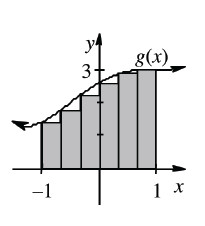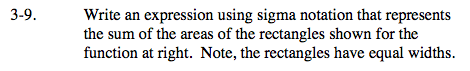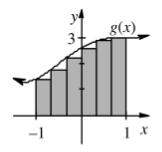### Home > CALC > Chapter Ch3 > Lesson 3.1.1 > Problem3-9

3-9.
1. Write an expression using sigma notation that represents the sum of the areas of the rectangles shown for the function below. Note, the rectangles have equal widths. Homework Help ✎Questions to consider:

How many rectangles are there, n?Are they left-endpoint, right-endpoint or midpoint rectangles?

Left-endpoint:

$\sum_{i=0}^{n-1}\Delta xf\left ( a+\Delta xi \right )$

Right-endpoint:

$\sum_{i=1}^{n}\Delta xf\left ( a+\Delta xi \right )$

Midpoint:

$\sum_{i=0}^{n-1}\Delta xf\left ( a+\frac{\Delta x}{2}i \right )$

What is the width/base of each rectangle, Δx?

$\Delta x=\frac{b-a}{n}$

What is the function?

What is the starting value, a?
On what interval is the area being approximated?